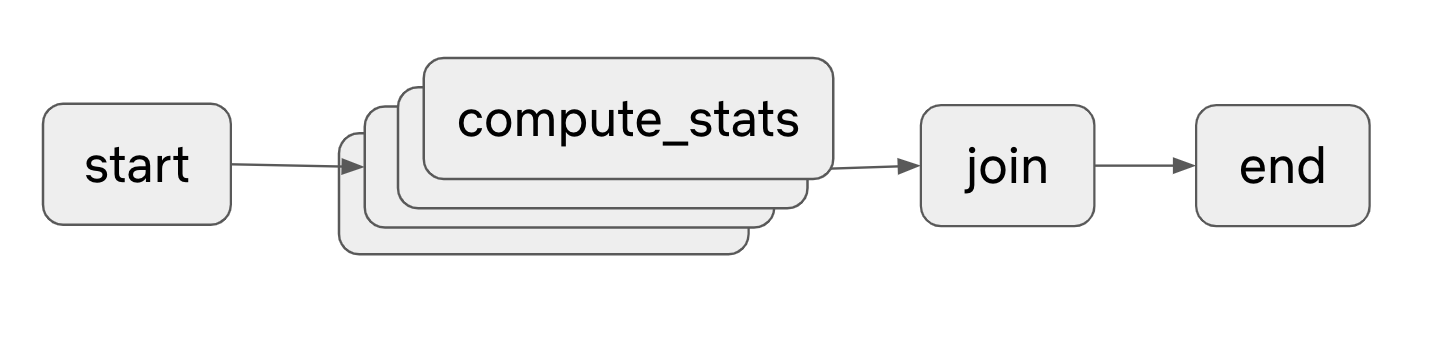# Episode 2: Statistics

Use Metaflow to load the movie metadata CSV file into a data frame and compute some movie genre specific statistics. These statistics are then used in later examples to improve our playlist generator.

You can optionally use the Metaflow client to eyeball the results in a Markdown Notebook, and make some simple plots.

## Showcasing:​

• Fan-out over a set of parameters using Metaflow foreach.
• Plotting results in a Markdown Notebook.

## To play this episode:​

If you haven't yet pulled the tutorials to your current working directory, you can follow the instructions here.

1. `cd tutorials/02-statistics`
2. `Rscript stats.R show`
3. `Rscript stats.R run`
4. Open `stats.Rmd` in RStudio

The dataset `movies.csv` is the same one as in Episode 01, which looks like this The dataset looks like this

movie_titletitle_yeargenregross
Avatar2009Sci-Fi760505847
Pirates of the Caribbean: At World's End2007Fantasy309404152
Spectre2015Thriller200074175
............The `MovieStatsFlow` below performs the following steps:

1. Ingests a CSV into a data frame.
2. Fan-out over genre using Metaflow foreach.
3. Compute median and mean for each genre.
4. Save a data frame of genre-specific statistics.
``library(metaflow)# The start step:start <- function(self){    # Loads the movie data into a data frame    self\$df <- read.csv("./movies.csv", stringsAsFactors=FALSE)    # find all unique genres    self\$genres <- levels(as.factor(self\$df\$genre))}# Compute statistics for a single genre.compute_stats <- function(self){    self\$genre <- self\$input    message("Computing statistics for ", self\$genre)    # Find all the movies that have this genre    self\$df_by_genre <- self\$df[self\$df\$genre == self\$genre, ]    gross <- self\$df_by_genre\$gross    # Get some statistics on the gross box office for these titles.    self\$median <- median(gross)    self\$mean <- mean(gross)}#  Join our parallel branches and merge results into a data frame.join <- function(self, inputs){    self\$stats <- data.frame(        "genres" = unlist(lapply(inputs, function(inp){inp\$genre})),        "median" = unlist(lapply(inputs, function(inp){inp\$median})),        "mean" = unlist(lapply(inputs, function(inp){inp\$mean})))    print(head(self\$stats))}metaflow("MovieStatsFlow") %>%    step(step = "start",          r_function = start,          next_step = "compute_stats",          foreach = "genres") %>%    step(step = "compute_stats",         r_function = compute_stats,         next_step = "join") %>%    step(step = "join",         r_function = join,         next_step = "end",         join = TRUE) %>%    step(step = "end") %>%    run()``